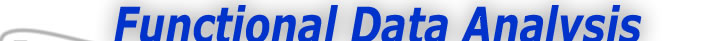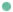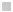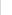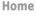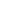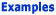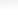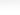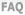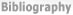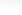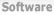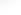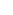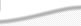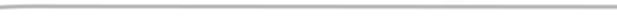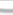BasisFunctions Goods Growth -What Data Am I working With -How do I define my B-spline basis? -What do we need to think about next? Weather
 From Raw Data to Functional Data Expertise: Beginner How do I define my B-spline basis? Two features must be specified. First, because B-splines are polynomial segments joined smoothly together, we must describe the degree of the polynomials segments, or, equivalently, their order = degree + 1. A good bet is to choose the order to be at least four larger than the highest order of derivative that you will need to look at. Here we will need to look at acceleration, or the second derivative, so we go with order 6, meaning that the B-splines are piecewise fifth degree polynomials. The second feature is a set of points called knots at which the these polynomials join. The first knot should be at or below the lowest age (one year old), and the last at or above the largest age (eighteen years old). The number of knots in between these plot the order determines the total number of basis functions. To keep things simple here, we will use the ages of observation themselves as knots. There are 31 ages, so the total number of B-spline basis functions is 29 + 6 = 35. In or Matlab software, the basis system is defined by the single command hgtbasis = create_bspline_basis([1,18], 35, 6, age); Sounds good. What's next? Now we can input the raw height measurements, the ages at which we made them, and the definition of the basis system into a function that computes the linear combination of the basis functions that best fits the data for each child. We organize our raw height measurements into a table with rows corresponding to ages of measurement, and columns corresponding to girls Here is a Matlab statement that does this for height measurements in a matrix hgtfmat: hgtffd = data2fd(hgtfmat, age, hgtbasis); The results are shown in Figure 1 for the first girl using the Matlab command plotfit_fd(hgtfmat, age, hgtffd);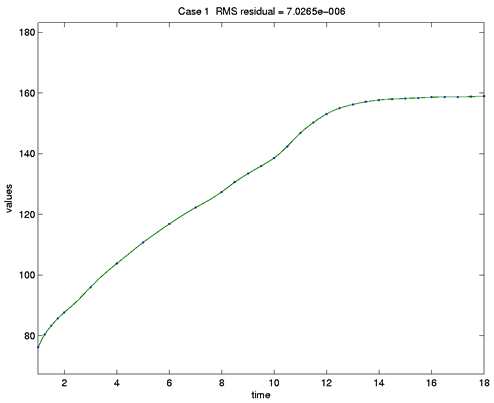Figure 1: The height of the first girl in the Berkeley Growth Study. Dots indicate the ages at which measurements were taken. The title indicates that the typical fitting error is about 0.0000007cm. No attempt is made here to deal with measurement error.